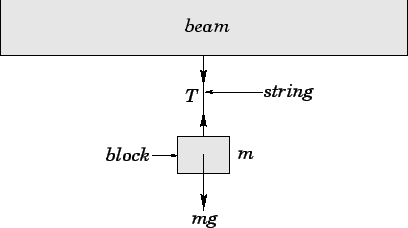# What are two ways all external force on an object are similar?

Jul 16, 2017
1. An object at rest on the earth

2. An object hanging from the ceiling on a string

#### Explanation:

I'll assume by the question what are two examples where all external forces on an object are equal in magnitude.

1.

One example where this is so is an object at rest on a horizontal surface on the earth.

The object has two forces acting on it:

• the downward force due to gravity (its weight)

• the upward normal force exerted by the surface

Here is the free-body diagram:The forces cancel out (because they are equal in magnitude and opposite in direction) so that the resultant is $0$, so it is at rest.

2.

Another instance is when an object is hanging on a string from a ceiling.

There are also two forces acting on the object:

• the downward gravitational force

• the upward tension force exerted by the string

Here is the free-body diagram:Same as the first example, the upward/downward forces cancel out, so the object is in equilibrium.